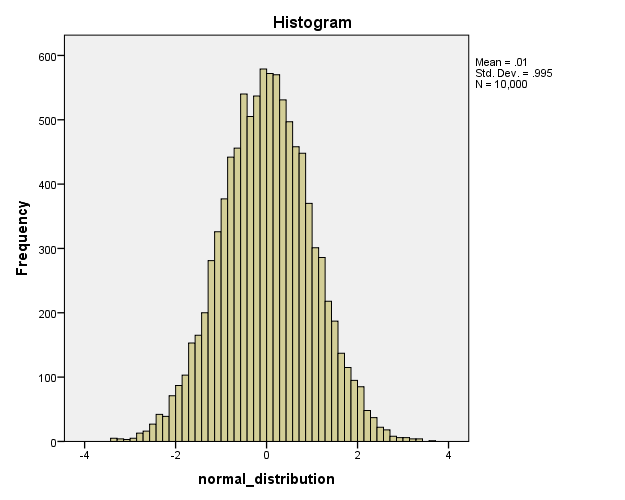SPSS TUTORIALS BASICS DATA ANALYSIS REGRESSION ANOVA T-TESTS

# SPSS RV Function – Quick Tutorial

## Basic Use

COMPUTE v1 = RV.NORMAL(0,1).

## Summary

In SPSS, `RV` is short for random variable. It draws random values from a given probability distribution.Strictly, probability density functions should be distinguished from probability distribution functions but we'll refer to both as “probability distributions”. The latter is specified as a period separated suffix as in `RV.BERNOULLI(.5)`.RV is mostly but not necessarily used with COMPUTE.Random Sample (N = 10,000) from a Standard Normal Distribution

## SPSS Rv Examples

A nice way to get familiar with some common probability distributions is to visualize them. The syntax below does this by computing random variables from different distributions and creating their histograms with FREQUENCIES. After running it, we'll briefly walk through the distributions we visualized.

## SPSS Rv Syntax Examples

*1. Create cases.

input program.
loop id = 1 to 1e4. /* 1e4 is short for 1 * 10**4 (= 10,000).
end case.
end loop.
end file.
end input program.

*2. Normal (Gaussian) distribution.

compute normal_distribution = rv.normal(0,1).

frequencies normal_distribution/histogram/format notable.

*3. Uniform distribution.

compute uniform_distribution = rv.uniform(0,5).

frequencies uniform_distribution/histogram/format notable.

*4. Bernoulli distribution.

compute bernoulli_distribution = rv.bernoulli(.3).

frequencies bernoulli_distribution/histogram/format notable.

*5. Binomial distribution.

compute binomial_distribution = rv.binom(5,.5).

frequencies binomial_distribution/histogram/format notable.

## Common Probability Distributions

• `RV.NORMAL(mean,SD)` is used for drawing values from a Gaussian ("normal") distribution. `RV.NORMAL(0,1)` returns random values from the standard normal distribution.
• `RV.UNIFORM(mininum,maximum)` draws values from a (continuous) uniform distribution. This means that all values have the same chance of occurring. An example are throws with a balanced die: values 1 through 6 all have the same chance of (1/6) of occurring. This can be simulated by `TRUNC(RV.UNIFORM(1,7))`. The fractional values are discretized here by truncating them.
• `RV.BERNOULLI(probability)` results in only zeroes and ones where the probability denotes the probability of drawing a one. For simulating flips with a balanced coin, use `RV.BERNOULLI(.5)`.
• `RV.BINOM(attempts,probability)` returns the (discrete) number of “successes” given the probability of success and the number of attempts. An example is the number of heads that come up when 5 coins are flipped. The result can be anything from 0 through 5. Therefore, `RV.BINOM(5,.5)` returns numbers from 0 through 5 as if they resulted from this experiment.Note that `RV.BINOM(1,probability)` is equivalent to `RV.BERNOULLI(probability)`. We usually say that “the Bernoulli distribution is a special case of the binomial distribution”.

## Final Notes

• For more probability distribution functions, consult the command syntax reference under “Universals”.
• Related to the `RV` function is the `CDF` (Cumulative Distribution Function). This returns the cumulative probability given a test statistic value and probability distribution.
• Also related to the `RV` and `CDF` functions is `IDF` (Inverse Distribution Function). It returns the test statistic value, given a cumulative probability and probability distribution.
• You could write `NORMAL(1)` instead of `RV.NORMAL(0,1)` and `UNIFORM(1)` for `RV.UNIFORM(0,1)`. This notation was used in SPSS versions 5 and below and still works for backward compatibility. We find it not very readable or intuitive and therefore rather avoid it.

# Tell us what you think!

*Required field. Your comment will show up after approval from a moderator.

# THIS TUTORIAL HAS 2 COMMENTS:

•### By Rahmani on September 22nd, 2016

excellent

•### By Ruben Geert van den Berg on September 23rd, 2016

Thanks for the compliment! Hope you'll like some of the other tutorials too.